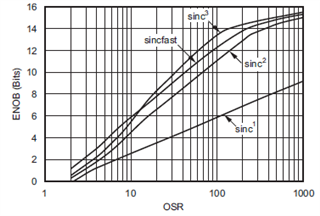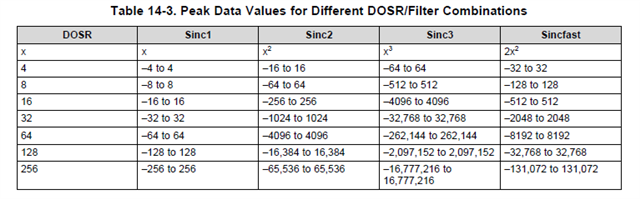If you have a related question, please click the "Ask a related question" button in the top right corner. The newly created question will be automatically linked to this question.

# TIDA-01606: Voltage conversion ratio and output data bit for delta-sigma modulator, sdfm.

Part Number: TIDA-01606
Other Parts Discussed in Thread: AMC1304M25, , TIDA-00080, AMC1306M25

Hello. I've been reading and understanding the documents provided for the delta-sigma modulator and SDFM.

But I still have doubts about my understanding.

First, I've been evaluating TIDA-00080 and TIDA-01606 for using AMC1304M25 with 28379d MCU for the current sensor.

Second, I realized the clipping voltage(+-320mV) and FSR(+-250mV) in the datasheet.

With this information, I'm going to use a 0.001Ω shunt resistor and measure the current up to +-200A. So it will not exceed the

range of FSR value in datasheet.

Third, I'm going to use 20MHz CLKIN clock frequency and 100 as OSR, SINC^3 filter.

So the datasheet of AMC1304M25 indicates the ENOB value is around 13-bit.Fourth, the datasheet indicates "(ENOB) depends upon Data filter type, DOSR, and sigma-delta modulator frequency."

So to increase or decrease the ENOB, with sinc^3 filter, 100 of DOSR, I need to change the modulator frequency.

Questions are as below.

1. The datasheet indicates using modulator(AMC1304M25, AMC1306M25)and 2837X MCU,

"device can achieve 16 bits of resolution with a dynamic range of 85 dB at a data rate of 78 kSPS."

But I couldn't find the information related to modulator frequency vs ENOB graph in the datasheet.

What information should I refer to expect the ENOB with filter type, DOSR, and modulator frequency?

2. Using given condition which is 20MHz modulator frequency, sinc^3 filter, 100 DOSR,

I can expect the value of filter output that can be read by 28379d MCU with table 14-3.Upper peak data value will be +1,000,000 and lower will be -1,000,000. It is around the 21-bit range.

But as I expected, ENOB will be 13-bit with given condition.

For this condition. What I understand is peak value, +-1,000,000 is just the maximum range.

If less than 21-bit data is entered, output data will be shown just like 13-bit data.

So. with 200A current goes 200mV for the input data of AINN and AINP.

250mV is maximum for that value so it is converted as 4096 so that 200mV is converted to around 3276.

So after I checked the output of the Data filter unit, then I can see the value of 3276 while in the real-time debugging panel.

But I think this assumption is not correct because, in the TIDA-01606 reference code, they normalize the filter data as dividing

the output filter data for 100^3(=DOSR^3).

I was wondering if you let me know whether my understanding is right or wrong.

If it is wrong with my think, please guide me the right way to expect the conversion ratio.

Thank you.

Best regards

Chun.

• Dear Chun,

1) I think that the modulator frequency is 20 MHz and the oversampling ratio is equal to 256. This will give you a data rate close to the mentioned one in the datasheet (78 kSPS). Based on the graph, the filter that has been used is the sinc 2.

2) If you select sinc 3 with a OSR equal to 100, you are going to have 100*100*100.

This will be equal to +1e6 -1e6 for the full scale range. This condition corresponds to  +312.5 mV -312.5 mV input analog voltage.

To get out the measured value, you need to apply the sequent formula: register output / 100*100*100 * 312.5 mV

To get out the per-unit value, you need to apply the sequent formula: register output / 100*100*100

To get out the current value, you need to apply the sequent formula: register output / 100*100*100 * 312.5 mV / 1e-3 Ohm